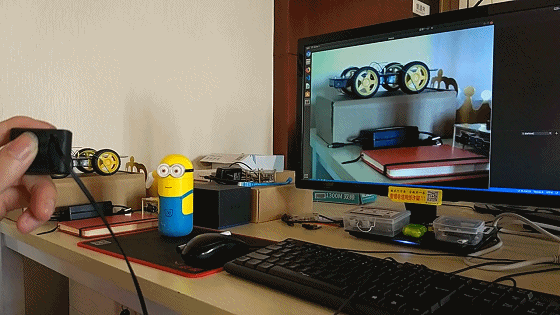Generating adversarial patches is as easy as drag and drop.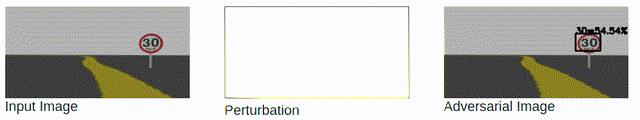Adversarial Attacks against End-to-End Object Detection

End-to-End deep learning models have been adopted in a variety of tasks.

End-to-End deep learning models have been adopted in a variety of tasks.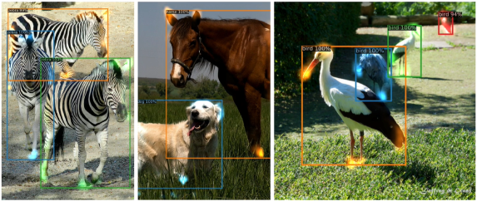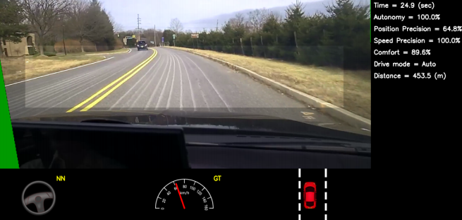End-to-End Autonomous Driving (NVIDIA)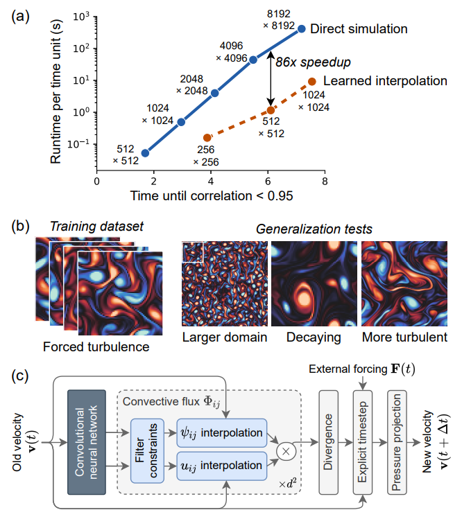However, deep neural networks are vulnerable to adversarial attacks.

Traning a model is like constructing a building.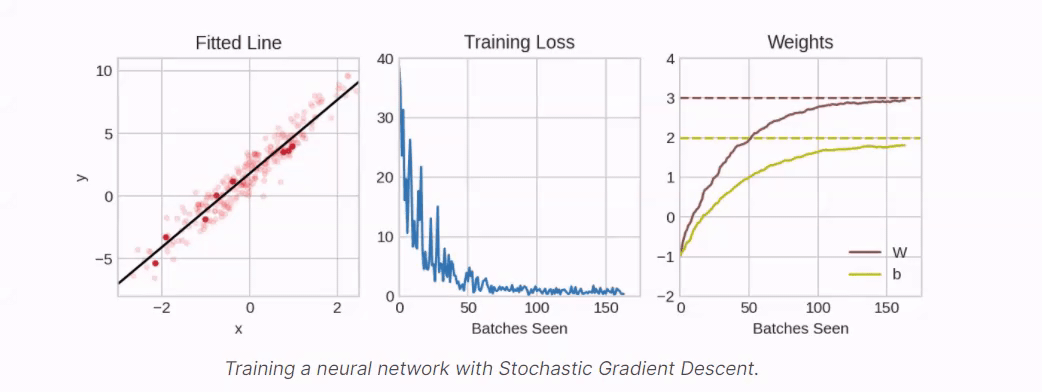Traning a model is like constructing a building.However, a small perturbation can detroy all.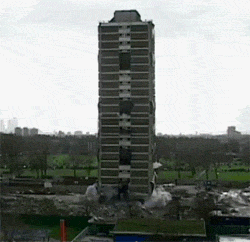Traning a model is like constructing a building.However, a small perturbation detroyes all.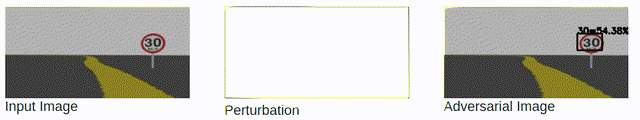## Full Demo

Deep Learning Models are differentiable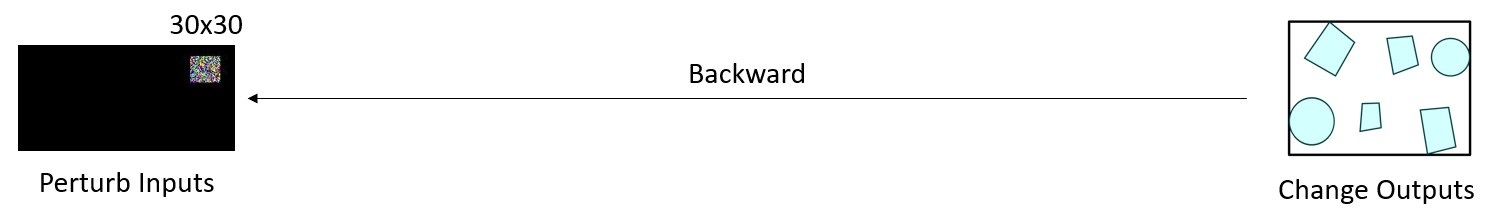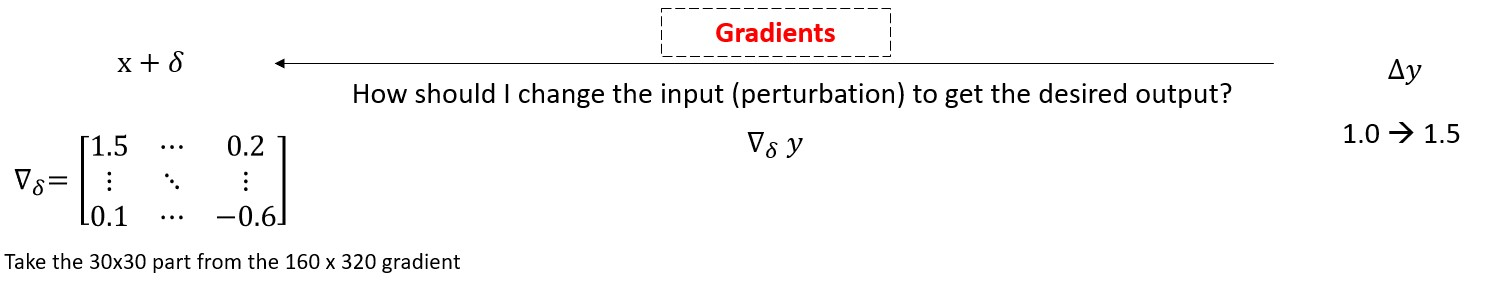Deep Learning Models are differentiable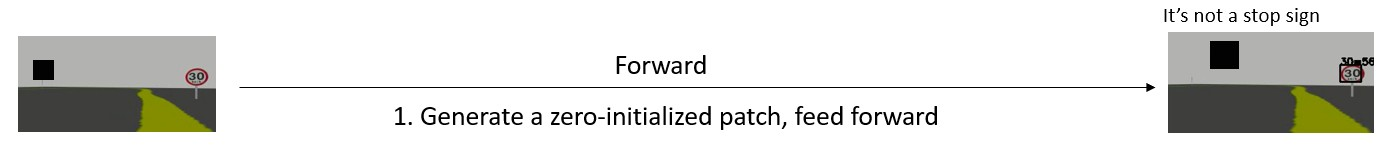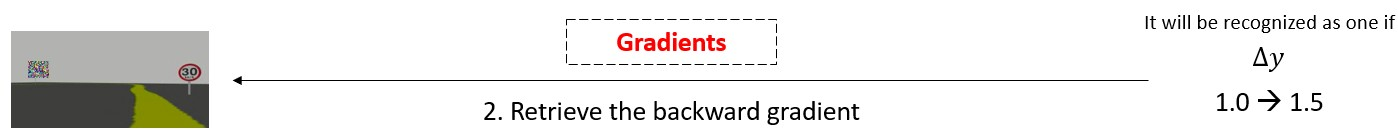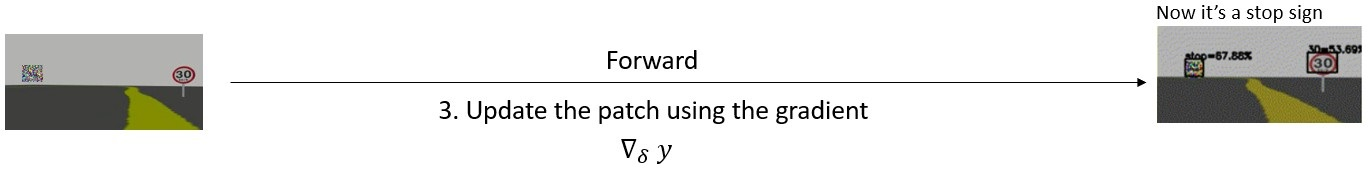Deep Learning Models are differentiableDeep Learning Models are differentiable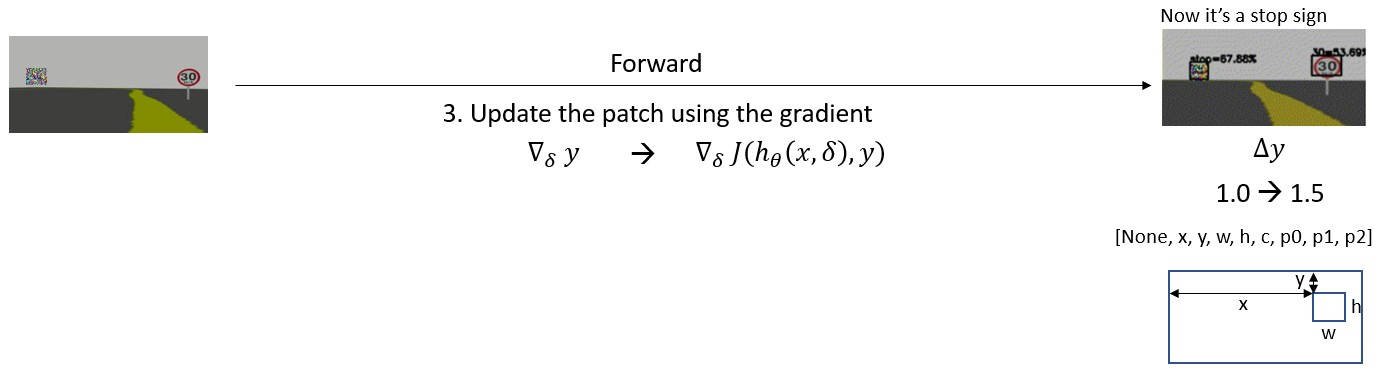Previous research usually calculates the gradient over the loss function.

\begin{aligned} \bigtriangledown_{\delta}J(h_{\theta}(x, \delta)), y) \end{aligned}

Deep Learning Models are differentiable

Previous research usually calculates the gradient over the loss function.

\begin{aligned} \bigtriangledown_{\delta}J(h_{\theta}(x, \delta)), y) \end{aligned}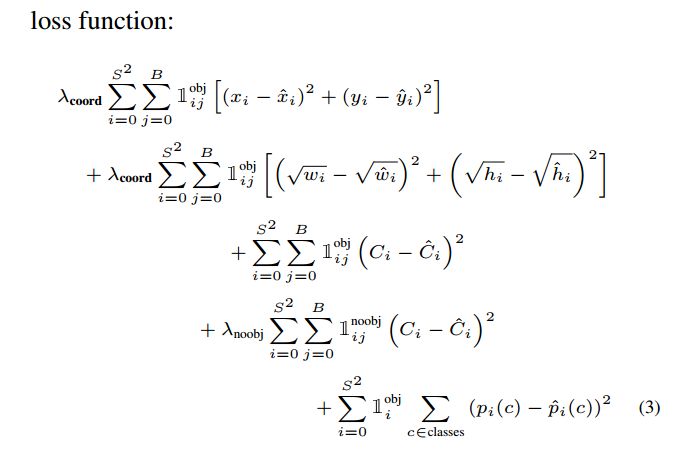• Calculating the loss function sometimes can be expensive. (slow iteration on the training set)
• For a real-time online attack, we don’t even know the ground truth. (infeasible to calculate the loss)

Deep Learning Models are differentiableIf we cannot use the loss function, what should we do?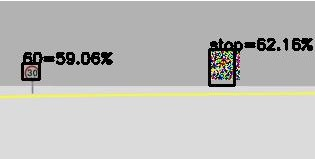One Targeted Attack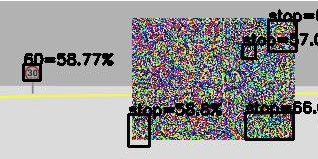Multi Targeted Attack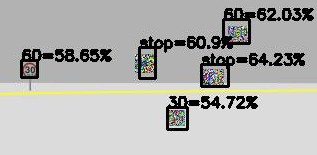Multi Untargeted Attack

$$J_1(x, \delta, y_h) = max(\ \sigma(c) * \sigma(p_0) \ )$$

                        
# One Targeted Attack
loss = K.max(K.sigmoid(K.reshape(out, (-1, 8))[:, 4]) * K.sigmoid(K.reshape(out, (-1, 8))[:, 5]))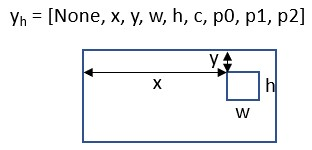One Targeted Attack

$$J_1(x, \delta, y_h) = max(\ \sigma(c) * \sigma(p_0) \ )$$

$$J_2(x, \delta, y_h) = \sigma(c) * \sigma(p_0)$$

                        
# Multi Targeted Attack
loss = K.sigmoid(K.reshape(out, (-1, 8))[:, 4]) * K.sigmoid(K.reshape(out, (-1, 8))[:, 5])Multi Targeted Attack

$$J_1(x, \delta, y_h) = max(\ \sigma(c) * \sigma(p_0) \ )$$

$$J_2(x, \delta, y_h) = \sigma(c) * \sigma(p_0)$$

$$J_3(x, \delta, y_h) = \sigma(c) * \sum\sigma(p_i)$$

                        
# Multi Untargeted Attack
loss = K.sigmoid(K.reshape(out, (-1, 8))[:, 4]) * K.sum(K.sigmoid(K.reshape(out, (-1, 8))[:, 5:]))Multi Untargeted Attack

#### Weakness: fast but not the best

Object Detection in real world